• 参数与返回值
• 重载方法
• 构造函数与方法链
• 析构函数

``````[<访问修饰符>] <返回类型> <方法名> ([<参数列表>])
{
// 方法体
}
``````

• <访问修饰符>可以定义方法的访问级别，包含常用的private、public、protected、internal，以及定义静态方法的static关键字、抽象方法的abstract关键字、虚拟方法的virtual关键字和重写方法的override关键字。如果没有访问修饰符，则默认为私有的访问级别。

• <返回值类型>是指方法执行后返回的数据类型，如前面使用过的int、string等类型，也可以是后续课程所介绍的各种类型，方法体中可以使用return语句返回执行结果数据。如果方法不需要返回数据，则使用void关键字设置。

• <方法名>当然是指方法的名称，如前面使用过的Drive()、Return()、ShowLocalModel()方法等。

• <参数列表>用于带入方法所需要的数据，如果没有就空着。参数可以有多个，每个参数最少需要定义数据类型和参数名称，多个参数使用英文半角逗号分隔；稍后，我们会详细讨论参数的应用问题。

• 方法体是方法真正实现功能的地方，使用一对花括号定义，这也是语句块的定义方式。

参数与返回值

普通参数

``````namespace ConsoleTest
{
public class C5
{
public static int Add(int x, int y)
{
return x + y;
}
}
}
``````

``````using System;

namespace ConsoleTest
{
class Program
{
static void Main(string[] args)
{
int x = 10;
int y = 99;
Console.WriteLine("{0} + {1} = {2}", x, y, C5.Add(x, y));
}
}
}
``````参数默认值

``````public static int Add1(int x, int y = 0)
{
return x + y;
}
``````

``````using System;

namespace ConsoleTest
{
class Program
{
static void Main(string[] args)
{
}
}
}
``````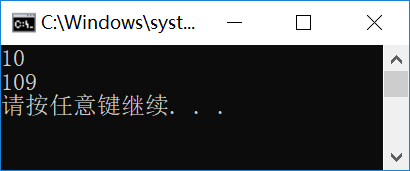参数数组

``````public static int Add2(int x, params int[] nums)
{
int sum = x;
for (int i = 0; i < nums.Length; i++)
sum = sum + nums[i];
return sum;
}
``````

``````params int[] nums
``````

``````using System;

namespace ConsoleTest
{
class Program
{
static void Main(string[] args)
{
}
}
}
``````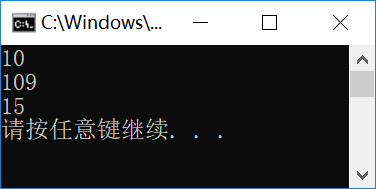ref参数

C#中的数据类型分为值类型和引用类型，它们在内存中的处理方式是不同的，但在直接应用时，表面上可能不太容易看出它们的区别，所在，这里着重讨论按值传递和按引用传递区别。

``````using System;

namespace ConsoleTest
{
class Program
{
static void Main(string[] args)
{
int x = 10;
int y = x;
Console.WriteLine("x={0},y={1}",x, y);
x = 99;
Console.WriteLine("x={0},y={1}", x, y);
}
}
}
``````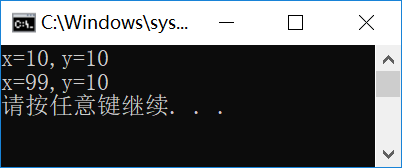int是值类型，而各种class类型则是引用类型，下面的代码，我们看一下CAuto类型的赋值操作。

``````static void Main(string[] args)
{
CAuto racer = new CAuto()
{
DoorCount = 2
};
CAuto suv = racer;
Console.WriteLine("{0},{1}", racer.DoorCount, suv.DoorCount);
suv.DoorCount = 5;
Console.WriteLine("{0},{1}", racer.DoorCount, suv.DoorCount);
}
``````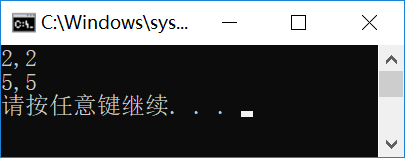``````public static void Swap(ref int x, ref int y)
{
int z = x;
x = y;
y = z;
}
``````

``````static void Main(string[] args)
{
int x = 10;
int y = 99;
Console.WriteLine("x={0},y={1}",x, y);
C5.Swap(ref x, ref y);
Console.WriteLine("x={0},y={1}", x, y);
}
``````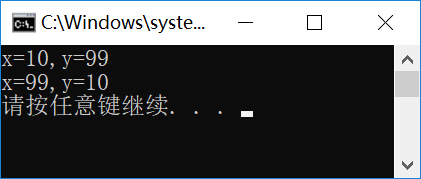out参数与返回值

``````static void Main(string[] args)
{
string s = "123";
int num = 0;
bool result = int.TryParse(s, out num);
Console.WriteLine("转换结果：{0}", result);
Console.WriteLine("转换数据：{0}", num);
}
``````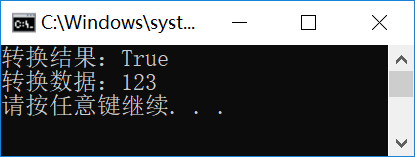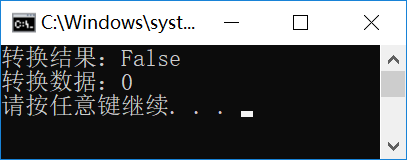``````public static bool TryToInt(object o, out int result)
{
if (o == null)
{
result = 0;
return false;
}
else
{
return int.TryParse(o.ToString(), out result);
}
}
``````

``````static void Main(string[] args)
{
object obj = "abc";
int num = 0;
bool result = C5.TryToInt(obj, out num);
Console.WriteLine("转换结果：{0}", result);
Console.WriteLine("转换数据：{0}", num);
}
``````

重载方法

``````//
public static void Swap(ref long x, ref long y)
{
long z = x;
x = y;
y = z;
}
//
public static void Swap(ref decimal x, ref decimal y)
{
decimal z = x;
x = y;
y = z;
}
``````

构造函数与方法链

``````public class CAuto
{
// 构造函数
public CAuto(int doorCount,string model)
{
DoorCount = doorCount;
Model = model;
}
//
public CAuto(string model) : this(4, model) { }
//
public CAuto() : this("未知型号") { }
//
}
``````

``````static void Main(string[] args)
{
CAuto car1 = new CAuto(2, "Coupe");
CAuto car2 = new CAuto("CX5");
CAuto car3 = new CAuto();
Console.WriteLine("{0},{1}", car1.DoorCount, car1.Model);
Console.WriteLine("{0},{1}", car2.DoorCount, car2.Model);
Console.WriteLine("{0},{1}", car3.DoorCount, car3.Model);
}
``````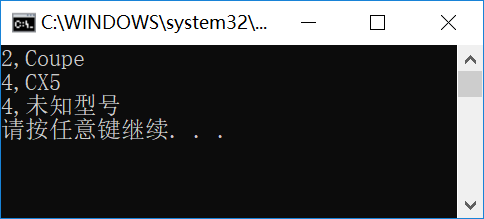析构函数

``````// 析构函数
~CAuto()
{
Console.WriteLine("汽车对象回收中");
}
``````

``````static void Main(string[] args)
{
CAuto car = new CAuto();
//car = null;
}
``````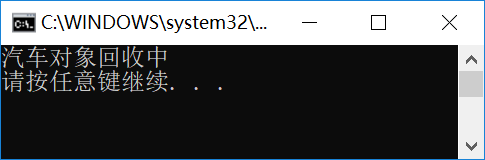CHY软件小屋原创作品！